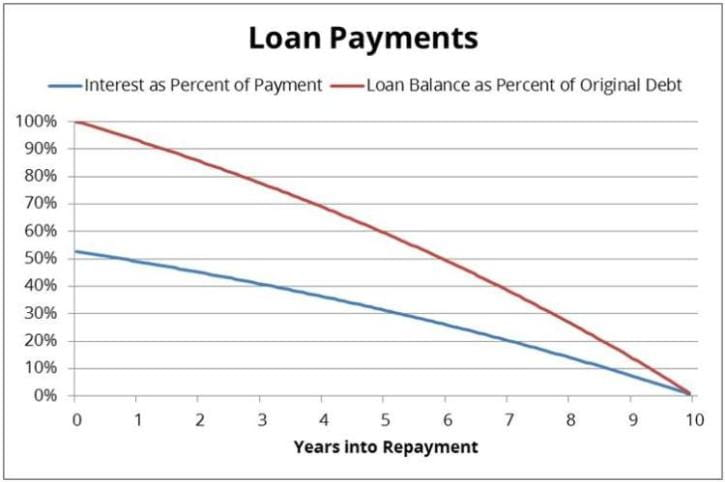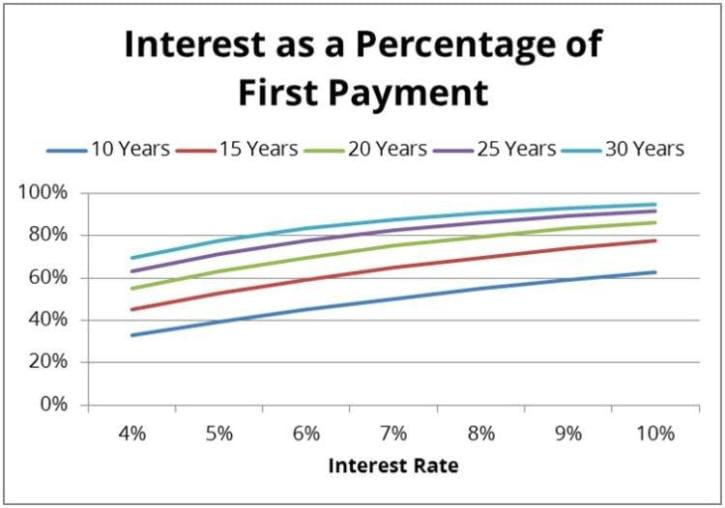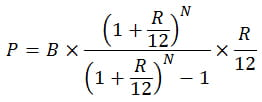# Understanding Interest Rates

Most borrowers don’t like paying interest. Many also don’t understand how interest works. Some of the more common issues concerning interest include:

• Complaints about most of a loan payment being applied to interest instead of principal (the amount borrowed)
• Complaints about paying back more in interest over the life of the loan than the original amount borrowed
• Complaints about not making much progress in paying down the principal balance of a loan, despite having been in repayment for several years
• Complaints about an interest rate being too high, especially when comparing fixed and variable interest rates
• Incorrect claims that doubling an interest rate will double the loan payments

If borrowers had a better understanding of how interest works, they might borrow less.

Find & Compare Private Student Loans for Your School:

CONTENTS:

## How Interest is Calculated

The interest on a student loan is calculated by multiplying the loan balance with the annual interest rate and the number of days since the last payment divided by the number of days in the year. Loan payments are applied first to interest, second to principal. This has several consequences:

• If the payment is less than the interest that has accrued since the last payment, the loan is said to be negatively amortized. If the unpaid interest is capitalized – added to the principal loan balance – interest will start being charged on the interest balance, not just the principal balance. This increases the cost of the loan faster and faster.
• Interest is charged every day on the unpaid principal balance, even if the obligation to make a payment has been temporarily suspended through a deferment or forbearance. (During a deferment, the federal government will pay the interest as it accrues on subsidized loans. The government does not pay the interest on unsubsidized loans during a deferment or on any loans during a forbearance.)
• Interest continues to accrue during periods of nonpayment, and can cause the loan balance to grow significantly during an extended period of nonpayment. In particular, interest continues to be charged when a borrower is late with a payment or stops repaying the loan.
• If a payment is received late, more interest will have accrued, so less of the payment will be applied to the principal balance of the loan.
• Reducing the loan payments by using an alternate repayment plan means that less of each payment will be applied to paying down the principal balance of the loan. Loan payments are applied first to interest, and any remaining money is applied to the principal balance.
• A borrower who is in a 20-year repayment term but chooses to make higher monthly payments every month that are the same as a borrower in a 10-year repayment term will pay off the loan in 10 years. There effectively is no difference between this borrower and a borrower with the same debt in a 10-year repayment term. It doesn’t matter whether the borrower is in a 10-year, 15-year, 20-year, 25-year or 30-year repayment term; if the borrower is making the same payments as a borrower in a 10-year repayment term, the borrower is effectively in a 10-year repayment term.
• Interest is charged from the date the loan is ^&*disburse*&^d. For example, a significant amount of interest may accrue on an unsubsidized federal student loan while the student is enrolled in college. Since payments are applied first to interest, the borrower’s loan payments must first pay off the accumulated interest balance before there will be any progress in paying down the principal balance of the loan. The sum of the principal balance and the accrued but unpaid interest will exceed the original amount borrowed until the accumulated interest has been paid off. To measure progress in paying off a debt, compare the current loan balance (sum of the principal and interest balances) with the loan balance when the loan entered repayment.
• Even after the accumulated in-school interest has been paid off, most of the initial loan payments will go to the new interest, as opposed to the principal balance. Interest is charged on the principal balance, which is highest at the start of repayment. As the borrower makes payments on the loan, the principal balance will decrease, causing the new interest that accrues between payments to decrease, so more of each payment will be applied to the principal balance. Progress in paying down the principal balance of a loan is quicker as the end of the repayment term approaches.
• Reducing the monthly payment by increasing the repayment term or duration of the loan will slow progress in paying down the principal balance. The loan payments will still be applied first to the new interest that accrued, so the smaller monthly payment means a smaller reduction in the principal balance of a loan. The principal balance will persist at higher levels for a longer time, increasing the total interest paid over the life of the loan. For example, increasing the repayment term on a 7% loan from 10 years to 20 years cuts the monthly payment by a third, but more than doubles the total interest paid over the life of the loan.

## Repayment Trajectory

To illustrate the progress in repaying a loan, consider this loan payment chart, which is based on a 10-year loan at 7.5% interest. Initially, more than half of each loan payment is applied to interest and the rest to principal. By the end of year 4, about 37% of each loan payment is applied to interest. By the end of year 7, only 21% of each loan payment is applied to interest.

The progress in paying down the principal balance of the debt accelerates as the end of the repayment term approaches. During the first year, only 6% of the original debt is paid down. This increases to 9% during the fourth year, 11% during the seventh year and 14% during the final year.The next chart shows how the interest as a percentage of the first payment increases with the interest rate and increases with the repayment term. This means less of each payment will be applied to paying down the principal balance of the loan.## Common Interest and Loan Payment Calculation Errors

Consider a \$10,000 loan with a 10-year repayment term and a 7% fixed interest rate. On financial literacy and math quizzes, when students and parents are asked how much the borrower will pay over the life of the loan, the most common incorrect answers include:

• \$700 (7% of \$10,000). Interest is charged every year of the loan, not just the first year of the loan. Also, total payments include repayment of the principal balance of the loan, not just the interest.
• \$10,000. Total payments over the life of the loan include both interest and principal, not just principal.
• \$10,700. This figure adds one year of interest to the principal balance, but fails to include interest paid during the rest of the loan term.
• \$7,000 (7% of \$10,000 multiplied by 10). This figure attempts to calculate the interest paid over the life of the loan, but fails to consider that the principal balance decreases each year in repayment, reducing the interest charged each year. It also fails to include repayment of the principal balance of the loan.
• \$17,000. This answer makes the same error in calculating the interest over the life of the loan, but does remember to include repayment of the principal balance of the loan.

The correct answer is \$13,933, which includes the \$10,000 principal balance of the loan and \$3,933 in interest.  One could use a loan payment calculator to determine the monthly payment and total interest paid, or one could solve a set of nonlinear equations to determine thatwhere P is the monthly payment, B is the loan balance, R is the interest rate and N is the number of payments in the repayment term. Perhaps, a simpler approach is to use a good rule of thumb for estimating the total interest, which is to approximate the average annual interest by dividing the first year’s interest by two. Multiply the average annual interest by the number of years in the repayment term to obtain a slight underestimate of the total interest paid over the life of the loan. For example, dividing 7% of \$10,000 in half yields \$350, and multiplying this by 10 years yields \$3,500 in total interest over the life of the loan, only slightly lower than the actual figure.

## Comparing Fixed and Variable Interest Rates

Another common source of confusion is the difference between fixed and variable interest rates. A fixed interest rate is the same for the life of the loan. This yields the same loan payment every month in the standard or extended repayment plans, both of which are level repayment plans. A variable interest rate may change throughout the life of the loan, yielding a different loan payment every time the interest rate changes. The interest rate when the loan enters repayment may be much different than the interest rate when the borrower first obtained the loan.

Also, variable interest rates are often expressed as the sum of a variable rate index and a fixed margin. The variable rate index is a reference rate that changes periodically, such as the LIBOR index, the Prime Lending Rate or the interest rates on U.S. Treasuries. For example, the lender might specify the interest rate as Prime + 5%. Some borrowers may misinterpret this as a 5% fixed interest rate, ignoring the variable-rate index part of the equation. If the Prime Lending Rate is 3.25%, then Prime + 5% yields an 8.25% interest rate. If the Prime Lending Rate were to increase to 6%, then Prime + 5% would yield an 11% interest rate. Variable interest rates typically change monthly, quarterly or annually.

It is important for borrowers to know and understand how interest rates will be calculated before borrowing loan funds.